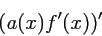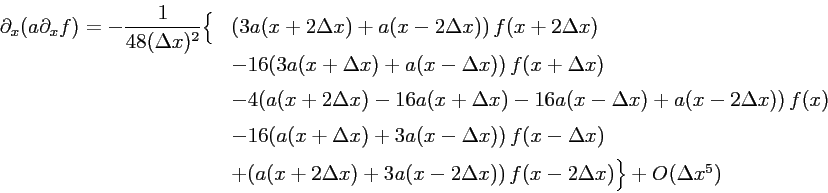### Implementation - Nothing is simple

• Finite differences:
• numerical solution based on DeRaedt's algorithm
• starting point: discrete version of ∂x2 to 4th order
•• PML-enhanced Schrödinger equation → discrete version of
•• where
•• one possible solution
•• how can one find it?
• general ansatz containing 25 terms
• symmetry Δx → -Δx ⇒ 13 terms
• expand it into a taylor series and compare to the desired result (MuPad)
• → singular linear system for the coefficients
• systematical scan of its solution space with Matlab →
• 43 formulae with 13 terms
• 15 formulae with 12 terms
• no shorter ones
• Performance considerations:
• problems
• complicated formula
• coefficients a(x+nΔx) are depending on x - bad for DeRaedt algorithm
• number of grid points has to be enlarged, typically by factor 2
• first implementation: 22x slower than non-PML version
• hard work
• extensive caching of intermediate results
• fast library for submatrix computations
• optimisation of memory accesses
• result: 3.3x faster
• 6.5x slower than non-PML version
• factor 2 already due to larger grid